# Utility Billing FAQ

Open as: PDF or Word Document

## 7. Rate Maintenance

Rate Code Maintenance

Editing a Rate Code

"Flat" and "One Time" Rates

"Metered" Rates

"Stepped" or "Variable" Rates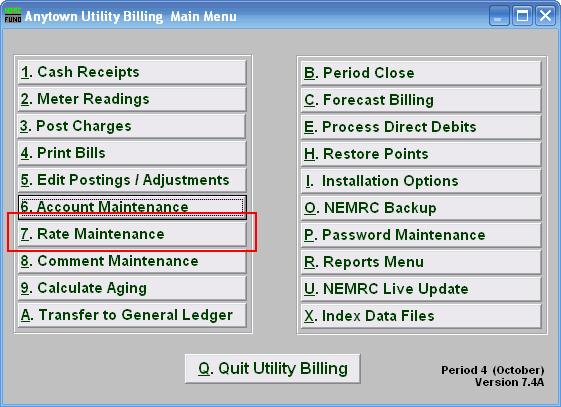Click on "7. Rate Maintenance" from the Main Menu, and the following window will appear:

Rate Code Maintenance

Choosing / Adding a Rate Code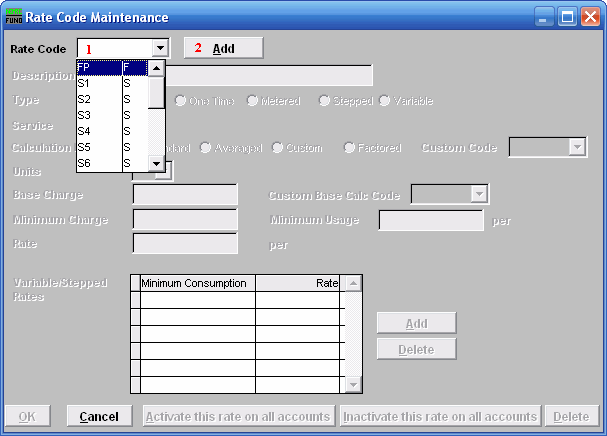1. Rate Code : To select from a previously established rate, click the drop down arrow, and move the mouse over the code representing the rate you wish to edit, and click on it.
2. Add : To create a new rate code, click "Add." The following window will appear: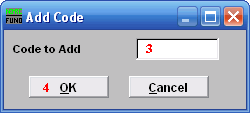3. Code to Add : Type in an alphanumeric code to add, maximum of four (4) characters.
4. Click "OK" to begin editing the new code.

Editing a Rate Code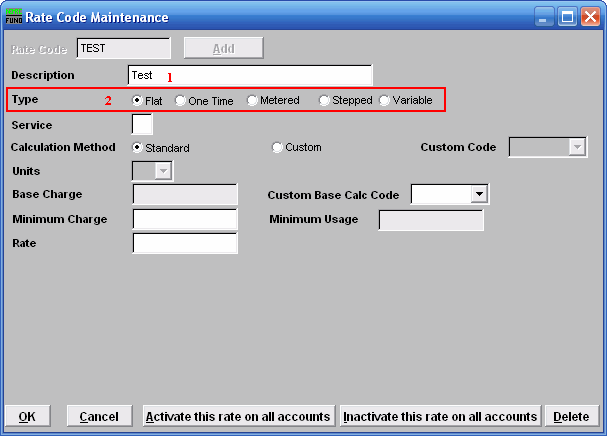1. Description : You MUST fill out a short description for this rate. This description will usually appear on the bill form.
2. Type : Click on the circle next to the type of rate that this rate is going to be. Refer to the section below that relates to your choice of Rate type.

"Flat" and "One Time" Rates

Flat : Unit is multiplied by a Rate.

One Time : Rate will automatically be removed from the customer account after printing the bill. Unit is multiplied by a Rate.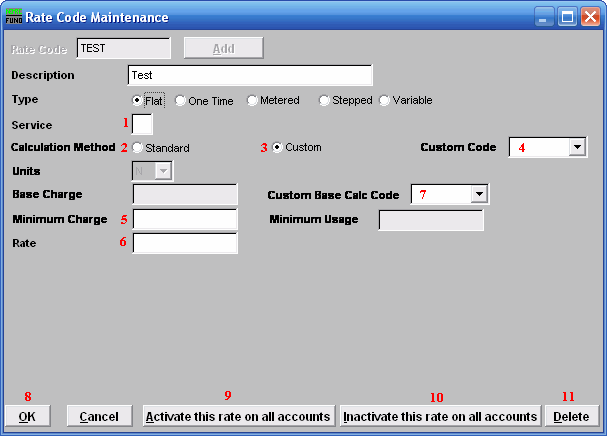1. Service : Type the single letter that represents what service this rate is for. Services are defined in "I. Installation" from the Main Menu.
2. Calculation Method - Standard : Uses the method described for the Type of Rate you chose. Standard is a straight formula calculation. Click the circle next to "Standard" to choose this option.
3. Calculation Method - Custom : A rate that is not standard, that NEMRC has created specifically for your use. Click the circle next to "Custom" to choose this option.
4. Custom Code : The code of the rate that NEMRC created for your use. This option is only available if you chose to use a Custom Calculation Method.
5. Minimum Charge : After calculation is done. If the total charge after calculation is less then the minimum charge, then the minimum charge will be billed to the customer.
6. Rate : This is the Rate per Unit. The amount to charge per unit as assigned in "6. Account Maintenance" in the "Rates" tab.
7. Custom Base Calc Code : A base calculation code created by NEMRC specifically for your use.
8. Click "OK" to save this rate and return to the Rate Code Maintenance window.
9. Click "Activate this rate on all accounts" to activate this rate on all accounts that have been assigned this rate code.
10. Click "Inactivate this rate on all accounts" to Inactivate this rate on all accounts that have been assigned this rate code.
11. Cick "Delete" to delete this rate.

"Metered" Rates

Metered : Billing amounts are calculated by evaluation of consumption.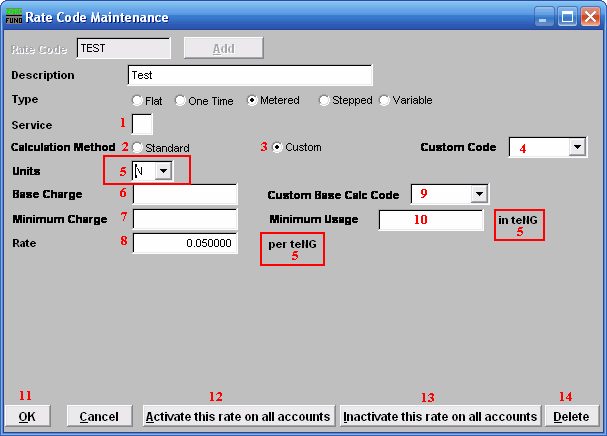1. Service : Type the single letter that represents what service this rate is for. Services are defined in "I. Installation" from the Main Menu.
2. Calculation Method - Standard : Uses the method described for the Type of Rate you chose. Standard is a straight formula calculation. Click the circle next to "Standard" to choose this option.
3. Calculation Method - Custom : A rate that is not standard, that NEMRC has created specifically for your use. Click the circle next to "Custom" to choose this option.
4. Custom Code : The code of the rate that NEMRC created for your use. This option is only available if you chose to use a Custom Calculation Method.
5. Units : Click the arrow to open the drop down list, and select the unit that you wish to use for this Rate. Meters don't need to read in the same units, the system will convert any meter units to rate units when it is necessary to calculate the amount to be charged. This also determines what the Rate is calculated on, and how the minimum usage is measured.
6. Base Charge : This amount will be charged, regardless of consumption. Flow is then calculated and added to this Base Charge.
7. Minimum Charge : After calculation is done. If the total charge after calculation is less then the minimum charge, then the minimum charge will be billed to the customer.
8. Rate : This is the Rate per Unit that was selected.
9. Custom Base Calc Code : A Base Calculation Code created by NEMRC specifically for your use.
10. Minimum Usage : If a meter doesn't register a volume equal to or greater then this, then this will be the volume that is used in the calculations of the bill.
11. Click "OK" to save this rate and return to the Rate Code Maintenance window.
12. Click "Activate this rate on all accounts" to activate this rate on all accounts that have been assigned this rate code.
13. Click "Inactivate this rate on all accounts" to Inactivate this rate on all accounts that have been assigned this rate code.
14. Click "Delete" to delete this rate.

"Stepped" or "Variable" Rates

Stepped : Billing amounts are calculated by evaluation of consumption. Each "step" is charged at its own rate, and added together for the total charge. May be increasing or decreasing rates.

Variable : Billing amounts are calculated by evaluation of consumption. Total usage is charged at the rate for the step reached.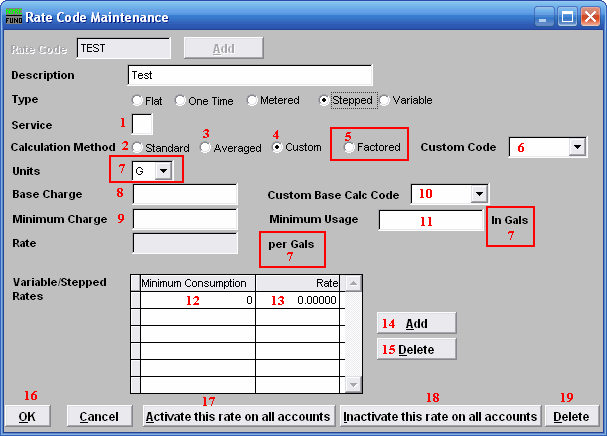1. Service : Type the single letter that represents what service this rate is for. Services are defined in "I. Installation" from the Main Menu.
2. Calculation Method - Standard : Uses the method described for the Type of Rate you chose. Standard is a straight formula calculation. Click the circle next to "Standard" to choose this option.
3. Calculation Method - Averaged : A calculation method where the consumption is first divided by the units entered for the rate in "6. Account Maintenance" in the "Rates" tab.
4. Calculation Method - Custom : A rate that is not standard, that NEMRC has created specifically for your use. Click the circle next to "Custom" to choose this option.
5. Calculation Method - Factored : A calculation method where the base charge and the minimum charge and the minimum consumptions are all multiplied by the rate in "6. Account Maintenance" in the "Rates" tab. Only available for "stepped" type rates.
6. Custom Code : The code of the rate that NEMRC created for your use. This option is only available if you chose to use a Custom Calculation Method.
7. Units : Click the arrow to open the drop down list, and select the unit that you wish to use for this Rate. Meters don't need to read in the same units, the system will convert any meter units to rate units when it is necessary to calculate the amount to be charged. This also determines what the Rate is calculated on, and how the minimum usage is measured.
8. Base Charge : This amount will be charged, regardless of consumption. Flow is then calculated and added to this Base Charge.
9. Minimum Charge : After calculation is done. If the total charge after calculation is less then the minimum charge, then the minimum charge will be billed to the customer.
10. Custom Base Calc Code : A base calculation code created by NEMRC specifically for your use.
11. Minimum Usage : If a meter doesn't register a volume equal to or greater then this, then this will be the volume that is used in the calculations of the bill.
12. Minimum Consumption : Whichever units that have been selected on the "Unit" field are used here. First "step" must start at zero (0). If the rate calculation method is factored, then the minimums will be multiplied by the units on this rate in "6. Account Maintenance" in the "Rates" tab.
13. Rate : The rate applied to consumption between the "step" before this, and the next "step" defined. If it is the last "step" then that rate is applied to the rest of consumption.
14. Add : Click "Add" and then start typing a new minimum consumption, and then click on the Rate field or hit the Enter key on your keyboard and type in the rate that is to be applied at that level of consumption.
15. Delete : Click "Delete" while a "step" is selected to delete that "step".
16. Click "OK" to save this rate and return to the Rate Code Maintenance window.
17. Click "Activate this rate on all accounts" to activate this rate on all accounts that have been assigned this rate code.
18. Click "Inactivate this rate on all accounts" to Inactivate this rate on all accounts that have been assigned this rate code.
19. Click "Delete" to delete this rate.